# Converting between λ and ν for Blackbody Radiation?!

Forgive me for this stupid question, but how do I convert between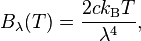and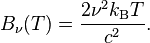I tried c = νλ but that doesn't work. This is the Rayleigh Jeans Law by the way.

Last edited:

Forgive me for this stupid question, but how do I convert between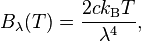andI tried c = νλ but that doesn't work.

pl. give the full expression of the quoted equation and where these two are being used.

The Rayleigh–Jeans law agrees with experimental results at large wavelengths (low frequencies) but strongly disagrees at short wavelengths (high frequencies). This inconsistency between observations and the predictions of classical physics is commonly known as the ultraviolet catastrophe,

see the full expressions
associated Rayleigh–Jeans limits are given by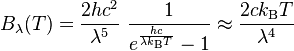or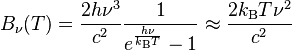now you can see the approximations- actually they are not exact expressions

vanhees71
Gold Member
2021 Award
You only have to remember that these are probability distributions ##\mathrm{d} N/\mathrm{d} \nu## or ##\mathrm{d} N/\mathrm{d} \lambda##. Now you have ##\nu=c/\lambda##. This implies
$$B_{\nu}=\frac{\mathrm{d} N}{\mathrm{d} \nu}=\frac{\mathrm{d} N}{\mathrm{d} \lambda} \left|\frac{\mathrm{d} \lambda}{\mathrm{d} \nu}\right| = B_{\lambda} \frac{c}{\nu^2}.$$
Now with
$$B_{\lambda}=\frac{2 c k_B T}{\lambda^4}=\frac{2 k_B T \nu^4}{c^3} \; \Rightarrow\; B_{\nu}=\frac{2 k_B T \nu^2}{c^2},$$
and this was to be shown.

Thank you guys.

Forandthe peak intensities occur at different wavelengths or frequencies.

How do scientists measure the spectral radiance of blackbodies? Are there TWO types of equipment, one for $$B_\lambda$$ and the other for $$B_\nu$$, such that each device yields a peak at a different frequency?